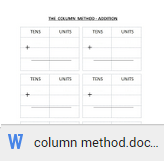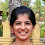Latest Articles

# Addition and Subtraction Strategies - Part 2

Info Post
In our previous post. we saw three methods under addition and subtraction strategies namely using pictures and counters, using the number line method and the 100 square grid method. Today, we will be seeing the other two methods namely the number bonds and the column method that is being used for solving maths sums in key stage 1.

## Method 4: The Number Bonds

The use of number bonds makes it easy for children to solve simple addition and subtraction quickly. It is an important mental strategy that is being introduced in key stage 1 and also continued later in a child's education. So, what are these number bonds? Number bonds are nothing but simple and basic pairing of numbers which is made familiar to the children by continuous practice. It helps children in doing quick mental addition.

For example,  3 + 2 = 5
In schools, number bonds up to 10 are practiced in Year 1 followed by number bonds up to 20. Download Number bonds worksheet to practice## Method 5: The Column Method

Column Method addition and subtraction are a conventional way of adding and subtracting that uses place values, which is by adding or subtracting 'units' digits together, 'tens' digits together and 'hundred' digits together. It can extends up to any number of digits but in key stage 1, the column method is used only for adding or subtracting two digit numbers which has tens and units.

Example:
From the above, we can see the numbers in a tabular form under units and tens. By column addition, we add the units digits together (1+5) and the tens digits together (5+4) to get our final answer (96). This method is often taught after learning about the other methods like using counters, tally method, number lines, grid method and number bonds.  Children are usually asked to use any of the methods that they learnt but number bonds method are the most preferred to solve column method.Download WorksheetDownload Worksheet

Hope the above methods gives you a fair idea of is taught in school. If you have any more methods to share, do drop a comment or mail me so that I know. Also, read Addition and Subtraction Strategies - Part 1.

Do you think Maths is not for you (or) say you can't handle Maths? Then, just spare a few minutes of your time on this link "What is Vedic Maths?". It will sure be informative :)

Share:

1.I loved the colour bond....that will be great for kids!

2.Maths always scare me but u make a good teacher with the color bond:)

3.Wonderful methods to learn maths! When I look back, I feel happy that our teacher were so good in teaching these basics! There were no charts I can recall even though they made us thorough in the basic maths... So nice of you sharing these tricks Uma! Thank you!

Thank You for visiting Momscribe.com !